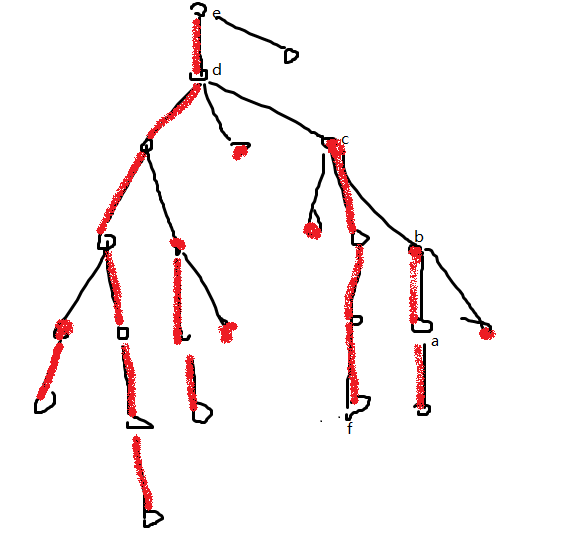# bzoj1758Wc10重建计划——solution

## 1758: [Wc2010]重建计划

Time Limit: 40 Sec  Memory Limit: 162 MB
Submit: 4707  Solved: 1200
[Submit][Status][Discuss]

## Description4
2 3
1 2 1
1 3 2
1 4 3

2.500

## HINT

N<=100000,1<=L<=U<=N-1,Vi<=1000000 新加数据一组 By leoly,但未重测..2016.9.27

-by bzoj
https://www.lydsy.com/JudgeOnline/problem.php?id=1758

check需要找条最长链，如果没有[L,U]的限制，可以直接DP最长链（最近怎么光见到这个）

(点分加单调队列应该可以nlog^2？？)

N^2枚举所有点，可以改成N^2枚举所有深度，然后可以用一个下标为深度的线段树变成NlogN——因为当两个深度中有一个确定后，另一个的范围是连续的一段区间

——把前i-1个儿子的子树和X合并，然后枚举第i个儿子的子树中的所有深度在X的线段树中查找(upd 2018.5.24:不采用线段树合并，转而采用基于长链剖分的暴力线段树插入，好像也可以保证效率)

#include<cstdio>
#include<cstring>
#include<algorithm>
#define LL long long
using namespace std;
const LL exp=10000;
const LL INF=1e15;
struct ss{
int to,next;
LL val;
}e;
int first,num;
struct DT{
LL max;
int ch;
}data;
int root,tot;
int dep,depst,hw;
LL val;
LL l,r,mid,ans;
int n,Low,Top;
void build(int ,int ,LL );
bool check(LL );
void dfs_1(int ,int );
void dfs_2(int );
void insert(int ,int ,int&,int ,LL );
LL get_max(int ,int ,int ,int ,int );
LL get_poi_max(int ,int ,int ,int );
void merge(int ,int ,int ,int&);
int main()
{
int i,j,k,o;
scanf("%d",&n);
scanf("%d%d",&Low,&Top);
for(i=1;i<n;i++){
scanf("%d%d%d",&j,&k,&o);
build(j,k,o*exp),build(k,j,o*exp);
}
dfs_1(1,0);
l=exp,r=1e10,mid=(l+r)>>1ll;
while(l<r-3){
if(check(mid))    l=mid;
else            r=mid-1;
mid=(l+r)>>1ll;
}
for(mid=r;mid>=l;mid--)
if(check(mid)){
printf("%.3lf",mid/10000.0);
return 0;
}
}
void build(int f,int t,LL v){
e[++num].next=first[f];
e[num].to=t,e[num].val=v;
first[f]=num;
}
bool check(LL lim){
int i;
memset(root,0,sizeof(root)),tot=0;
memset(data,0,sizeof(data)),data.max=-3*INF;
for(i=1;i<=num;i++)    e[i].val-=lim;
ans=-3e10;
dfs_2(1);
for(i=1;i<=num;i++)    e[i].val+=lim;
return ans>=0;
}
void dfs_1(int now,int fa){
int i;
dep[now]=dep[fa]+1;
depst[now]=dep[now],hw[now]=-1;
for(i=first[now];i;i=e[i].next)
if(e[i].to!=fa){
dfs_1(e[i].to,now);
if(depst[now]<depst[e[i].to])
depst[now]=depst[e[i].to],hw[now]=i;
}
}
void dfs_2(int now){
int i,j;
if(hw[now]!=-1){
val[e[hw[now]].to]=val[now]+e[hw[now]].val;
dfs_2(e[hw[now]].to);
root[now]=root[e[hw[now]].to];
if(dep[now]+Low<=depst[now]);
ans=max(ans,get_max(1,n,root[now],dep[now]+Low,min(depst[now],dep[now]+Top))-val[now]);
insert(1,n,root[now],dep[now],val[now]);
}
else{
insert(1,n,root[now],dep[now],val[now]);
return ;
}
for(i=first[now];i;i=e[i].next)
if(dep[e[i].to]>dep[now]&&i!=hw[now]){
val[e[i].to]=val[now]+e[i].val;
dfs_2(e[i].to);
for(j=dep[e[i].to];j<=depst[e[i].to]&&j<=dep[now]+Top;j++)
ans=max(ans,get_poi_max(1,n,root[e[i].to],j)-val[now]+get_max(1,n,root[now],max(dep[now]*2+Low-j,dep[now]+1),min(dep[now]*2+Top-j,depst[now]))-val[now]);
merge(1,n,root[e[i].to],root[now]);
}
}
void insert(int l,int r,int&now,int lim,LL x){
if(!now)now=++tot;
if(l==r){
data[now].max=x;
return ;
}
int mid=(l+r)>>1;
if(lim<=mid)
insert(l,mid,data[now].ch,lim,x);
else
insert(mid+1,r,data[now].ch,lim,x);
data[now].max=max(data[data[now].ch].max,data[data[now].ch].max);
}
LL get_max(int l,int r,int now,int L,int R){
if(L>R)return data.max;
if(L<=l&&r<=R)
return data[now].max;
int mid=(l+r)>>1;
LL lm=-INF,rm=-INF;
if(L<=mid)
lm=get_max(l,mid,data[now].ch,L,R);
if(R>mid)
rm=get_max(mid+1,r,data[now].ch,L,R);
if(lm>rm)    return lm;
return rm;
}
LL get_poi_max(int l,int r,int now,int lim){
if(l==r)return data[now].max;
int mid=(l+r)>>1;
if(lim<=mid)
return get_poi_max(l,mid,data[now].ch,lim);
else
return get_poi_max(mid+1,r,data[now].ch,lim);
}
void merge(int l,int r,int pre,int&now){
if(!now||!pre){
now+=pre;
return ;
}
if(l==r){
data[now].max=max(data[now].max,data[pre].max);
return ;
}
int mid=(l+r)>>1;
merge(l,mid,data[pre].ch,data[now].ch);
merge(mid+1,r,data[pre].ch,data[now].ch);
data[now].max=max(data[data[now].ch].max,data[data[now].ch].max);
}

（bzoj卡到39S......）

posted @ 2018-04-08 21:17  F.W.Nietzsche  阅读(285)  评论(0编辑  收藏  举报